Select Page

So far in our journey through Machine Learning with ML.NET, we used different machine learning algorithms to solve various tasks. Usually, at the end of each tutorial, we showed some metrics that determine how well the algorithms performed, but we haven’t explored that in more detail. In this article, we learn how we can measure the performance of machine learning algorithms and determine if we should do some improvements.

Are you afraid that AI might take your job? Make sure you are the one who is building it.

STAY RELEVANT IN THE RISING AI INDUSTRY! 🖖

## 1. Prerequisites

The implementations provided here are done in C#, and we use the latest .NET 5. So make sure that you have installed this SDK. If you are using Visual Studio this comes with version 16.8.3. Also, make sure that you have installed the following packages:

``\$ dotnet add package Microsoft.ML``

You can do the same from the Package Manager Console:

``Install-Package Microsoft.ML``

Note that this will install default Microsoft.ML package as well. You can do a similar thing using Visual Studio’s Manage NuGetPackage option: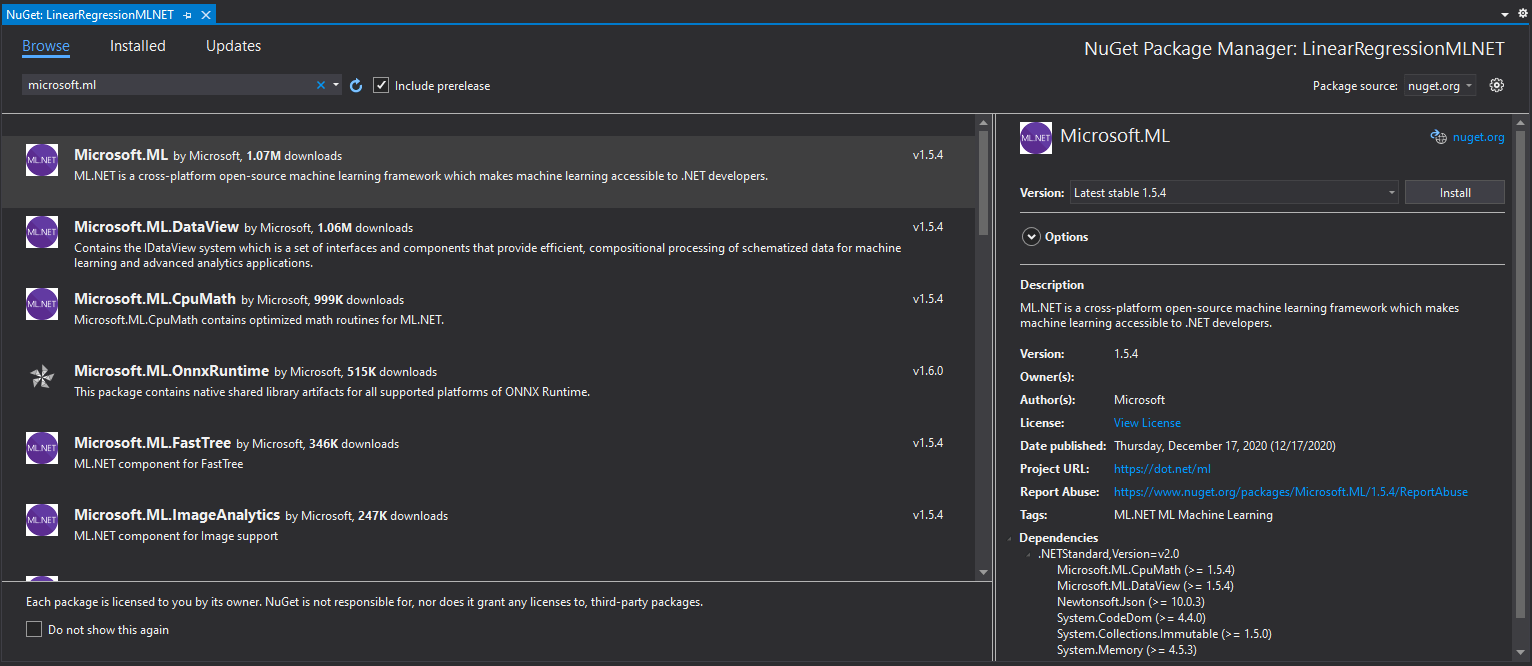If you need to catch up with the basics of machine learning with ML.NET check out this article

## 2. ML.NET Evaluation Metrics

In general, ML.NET groups evaluation metrics by the task that we are solving with some algorithm. This means that if we perform a binary classification task we use a different set of metrics to determine the performance of the machine learning algorithm, then when we perform the regression task.

Which makes sense. Binary classification tries to figure does sample belongs to a certain class, while regression in trying to model the continuous data. That is why we have to use different metrics to evaluate them.The MLContext class has different property (catalog) for every problem in the Machine Learning book. To continue with the previous example, the MLContext object has a property BinaryClassification which can be observed as a toolbox with all the things that you need for binary classification.

Each of these properties has a list of trainers, calibrators, etc. Each of them has methods Evaluate and EvaluateNonCalibrated, too. These methods return an object with metrics for a specific problem.  When we call MLContext.BinaryClassification.EvaluateNonCalibrated we get a BinaryClassificationMetrics object, which contains values of binary classification metrics.

Let’s explore each type of metric and see how we can measure the performance of machine learning algorithms with them.

## 3. Binary Classification Metrics

Since the problems we use in machine learning fall within different categories, we have different metrics for different types of problems. First, let’s explore metrics that are used for binary classification problems. In order to represent all these metrics we use simple data:

``````actual_values = [1, 1, 0, 1, 0, 0, 1, 0, 0, 0]
predictions =   [1, 0, 1, 1, 1, 0, 1, 1, 0, 0]``````

So our dataset is composed of two classes – Class 0 and Class 1. The model predicted the class of some samples well, while the others it misclassified.

### 3.1 Confusion Matrix

There are some basic terms that we need to consider when it comes to the performance of classification models. These terms are best described and defined through the confusion matrix. Confusion matrix or Error Matrix is one of the key concepts when we are talking about classification problems. This matrix is the NxN matrix and it is a tabular representation of model predictions vs. actual values.

Confusion Matrix is for the example data that we use is built like this:Each column and row is dedicated to one class. On one end we have the actual values and on the other end predicted values. What is the meaning of the values in the matrix? In the example above, from 4 values marked as Class 0, our model correctly classified 3 values and misclassified 1 value. This model, from the 6 values marked as Class 1, correctly labeled 3 and misclassified 3.

If we refer to Class 1 as positive and Class 0 as a negative class, then 3 samples predicted as Class 0 are called as true-negatives, and the 1 sample predicted as Class 1 is referred to as false-negative. The 3 samples correctly classified as Class 1 are referred to as true-positives, and those 3 misclassified instances are called false-positive.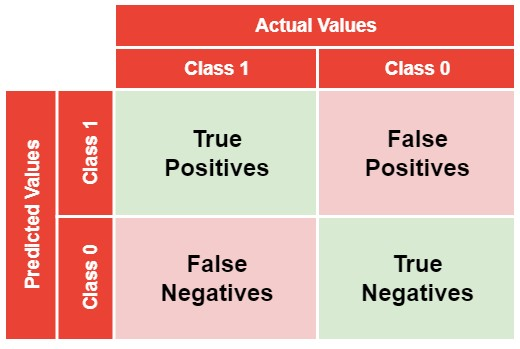Remember these terms, since they are important concepts in machine learning. It is one of the most asked questions in job interviews. Note that false-positives are also called Type I error and false-negatives are called Type II errors. The good news is that we can use ML.NET to get this matrix:

``````var mlContext = new MLContext();

var testSetTransform = trainedModel.Transform(dataSplit.TestSet);

var metrics = mlContext.BinaryClassification.EvaluateNonCalibrated(testSetTransform);

Console.WriteLine(metrics.ConfusionMatrix.GetFormattedConfusionTable());``````
``````TEST POSITIVE RATIO:    0.4608 (47.0/(47.0+55.0))
Confusion table
||======================
PREDICTED || positive | negative | Recall
TRUTH     ||======================
positive ||       46 |        1 | 0.9787
negative ||        2 |       53 | 0.9636
||======================
Precision ||   0.9583 |   0.9815 |``````

The result is exactly like in the table above. This matrix is not only giving us details about how our prediction model works but on the concepts laid out in this matrix we are building some of the other metrics. Some of them are Precision and Recall.

### 3.2 Precision

Precision is a very useful metric and it caries more information than the accuracy. Essentially, with precision, we answer the question: “What proportion of positive identifications was correct?”. It is calculated for each class separately with the formula:Its value can go from 0 to 1. It goes without saying that we should aim for higher precision, the closer to 1.00, the better.

### 3.3 Recall

Recall can be described as the ability of the classifier to find all the positive samples. With this metric, we are trying to answer the question: “What proportion of actual positives was identified correctly?” It is defined as the fraction of samples from a specific class which are correctly predicted by the model or mathematically:Precision and Recall are different and yet so similar. Precision is a measure of result relevancy, while recall is a measure of how many truly relevant results are returned. In the beginning, it might hard to decipher the difference between these two. If you are confused as well, imagine this situation.A client calls a bank in order to verify that her accounts are secure. Bank says that everything is properly secured, however in order to double-check, they ask a client to remember every time that she shared account details with someone. What is the probability that the client will remember every single time she did that?

So, the client remembers 10 situations in which that happened, even though in reality there were 8 situations, ie. she falsely identified two additional situations. This means that the client had a recall of 100%, meaning she did cover all the necessary options. However, the client’s precision is 80% since two of those situations are falsely identified as such.

### 3.4 Accuraccy

We start off with the metric that is easies one to understand – accuracy. It is calculated as a number of correct predictions divided by the total number of predictions. When we multiply it by 100 we get accuracy in percents.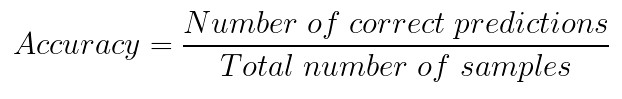To get the accuracy we use Accuracy of a BinaryClassificationMetrics object:

``````var mlContext = new MLContext();

var testSetTransform = trainedModel.Transform(dataSplit.TestSet);

var metrics = mlContext.BinaryClassification.EvaluateNonCalibrated(testSetTransform);

Console.WriteLine(\$"Accuracy: {metrics.Accuracy:0.##}");``````
``Accuracy: 0.97``

Accuracy however is a tricky metric because it can give us wrong impressions about our model. This is especially the case in the situations where the database is imbalanced, ie. there are many samples of one class and not much of the other. Meaning, if your model is performing well on the class that is dominant in the dataset, accuracy will be high, even though the model might not perform well in other cases. Also, note that models that overfit have an accuracy of 100%.

### 3.5 F1 Score

F1 Score is probably the most popular metric that combines precision and recall. It represents harmonic mean of them. For binarry classification, we can define it with formula: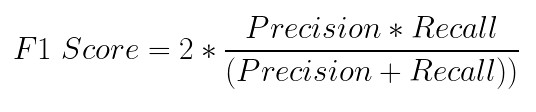To get F1 Score we use F1Score of a  BinaryClassificationMetrics object:

``````var mlContext = new MLContext();

var testSetTransform = trainedModel.Transform(dataSplit.TestSet);

var metrics = mlContext.BinaryClassification.EvaluateNonCalibrated(testSetTransform);

Console.WriteLine(\$"F1 Score: {metrics.F1Score:0.##}");``````
``F1 Score: 0.97``

### 3.6 Receiver Operating Characteristic (ROC) curve & Area Under the curve (AUC)

When predicting a class of a sample, the machine learning algorithm first calculates the probability that the processed sample belongs to a certain class and if that value is above some predefined threshold it labels is as that class.

For example, for a first sample algorithm predicts that there is a 0.7 (70%) chance that it is Class 0 and threshold is 0.6 the sample will be labeled as Class 0. This means that for different thresholds we can get different labels. This is where we can use the ROC (Receiver Operating Characteristic) curve. This curve shows the true positive rate against the false-positive rate for various thresholds.However, this metric isn’t helping us with model evaluation directly. What is expecially interesting about the image above is the area under the curve or AUC. This metric is, in fact, used as a measure of performance. We can say that ROC is a probability curve and AUC measure the separability, ie. AUC-ROC combination tells us our model capable to distinguish classes. The higher this value the better. We can use AreaUnderRocCurve of a BinaryClassificationMetrics object:

``````var mlContext = new MLContext();

var testSetTransform = trainedModel.Transform(dataSplit.TestSet);

var metrics = mlContext.BinaryClassification.EvaluateNonCalibrated(testSetTransform);

Console.WriteLine(\$"AUC-ROC: {metrics.AreaUnderRocCurve:0.##}");``````
``AUC-ROC: 1``

### 3.7 Area under Precision-Recall Curve (AUPRC)

In order to correctly evaluate a model, both metrics need to be taken into consideration. Unfortunately, improving precision typically reduces recall and vice versa. The precision-recall curve shows the tradeoff between precision and recall.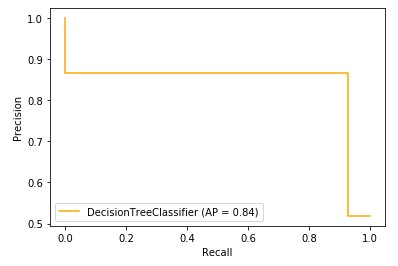The area under the curve represents both high recall and high precision. High scores for both show that the classifier is returning accurate results with a majority of positive results. To get this value we use AreaUnderPrecisionRecallCurve of a BinaryClassificationMetrics object.

``````var mlContext = new MLContext();

var testSetTransform = trainedModel.Transform(dataSplit.TestSet);

var metrics = mlContext.BinaryClassification.EvaluateNonCalibrated(testSetTransform);

Console.WriteLine(\$"AUPRC: {metrics.AreaUnderPrecisionRecallCurve:0.##}");``````
``AUC-ROC: 1.0``

## 4. Multi-Class Classification Metrics

For multi-class classification, we can use some of the same metrics that we use for binary classification. It is classification, after all. However, there are some specifics that are different for multi-class classification.

### 4.1 Confusion Matrix

Even if we perform multi-class classification, we can use a confusion matrix. The difference is that we have a row for each class. For example, if we use the NaiveBias algorithm for the classification of the Palmer Penguins Dataset, we can get something like this:

``````var mlContext = new MLContext();

var testSetTransform = trainedModel.Transform(dataSplit.TestSet);

var metrics = mlContext.MulticlassClassification.Evaluate(testSetTransform);

Console.WriteLine(modelMetrics.ConfusionMatrix.GetFormattedConfusionTable());``````
``````Confusion table
||========================
PREDICTED    ||     0 |     1 |     2 | Recall
TRUTH        ||========================
0.    Adelie ||    14 |    22 |    11 | 0.2979
1. Chinstrap ||     0 |    17 |     0 | 1.0000
2.    Gentoo ||     0 |     0 |    38 | 1.0000
||========================
Precision    ||1.0000 |0.4359 |0.7755 |``````### 4.2 Micro and Macro Accuracy

In general, we can apply an accuracy formula for binary classification for each class in the dataset. However, there are two ways to do that. We can treat all classes equally and compute the metric independently for each class and then take the average. This is called Macro Accuracy. The second approach would be to aggregate the contributions of all classes to compute the average metric. This is Micro Accuracy. Here is how to get these values with ML.NET:

``````var mlContext = new MLContext();

var testSetTransform = trainedModel.Transform(dataSplit.TestSet);

var metrics = mlContext.MulticlassClassification.Evaluate(testSetTransform);

Console.WriteLine(\$"Macro Accuracy: {modelMetrics.MacroAccuracy:#.##}{Environment.NewLine}" +
\$"Micro Accuracy: {modelMetrics.MicroAccuracy:#.##}{Environment.NewLine}");``````
``````Macro Accuracy: .77
Micro Accuracy: .68``````

Micro Accuracy is a more reliable metric since it suspects there might be a class imbalance, meaning there are have many more examples of one class than of other classes.

### 4.3 Log-Loss & Log-Loss Reduction

What is really interesting about this metric is that it is one of the most used evaluation metrics in Kaggle competitions. Log Loss is a metric that quantifies the accuracy of a classifier by penalizing false classifications. Minimizing this function can be, in a way, observed as maximizing the accuracy of the classifier.

Unlike the accuracy score, this metric’s value represents the amount of uncertainty of our prediction based on how much it varies from the actual label. For this, we need probability estimates for each class in the dataset. Mathematically we can calculate it as:Where N is the number of samples in the dataset, yi is the actual value for the i-th sample, and yi’ is a prediction for the i-th sample.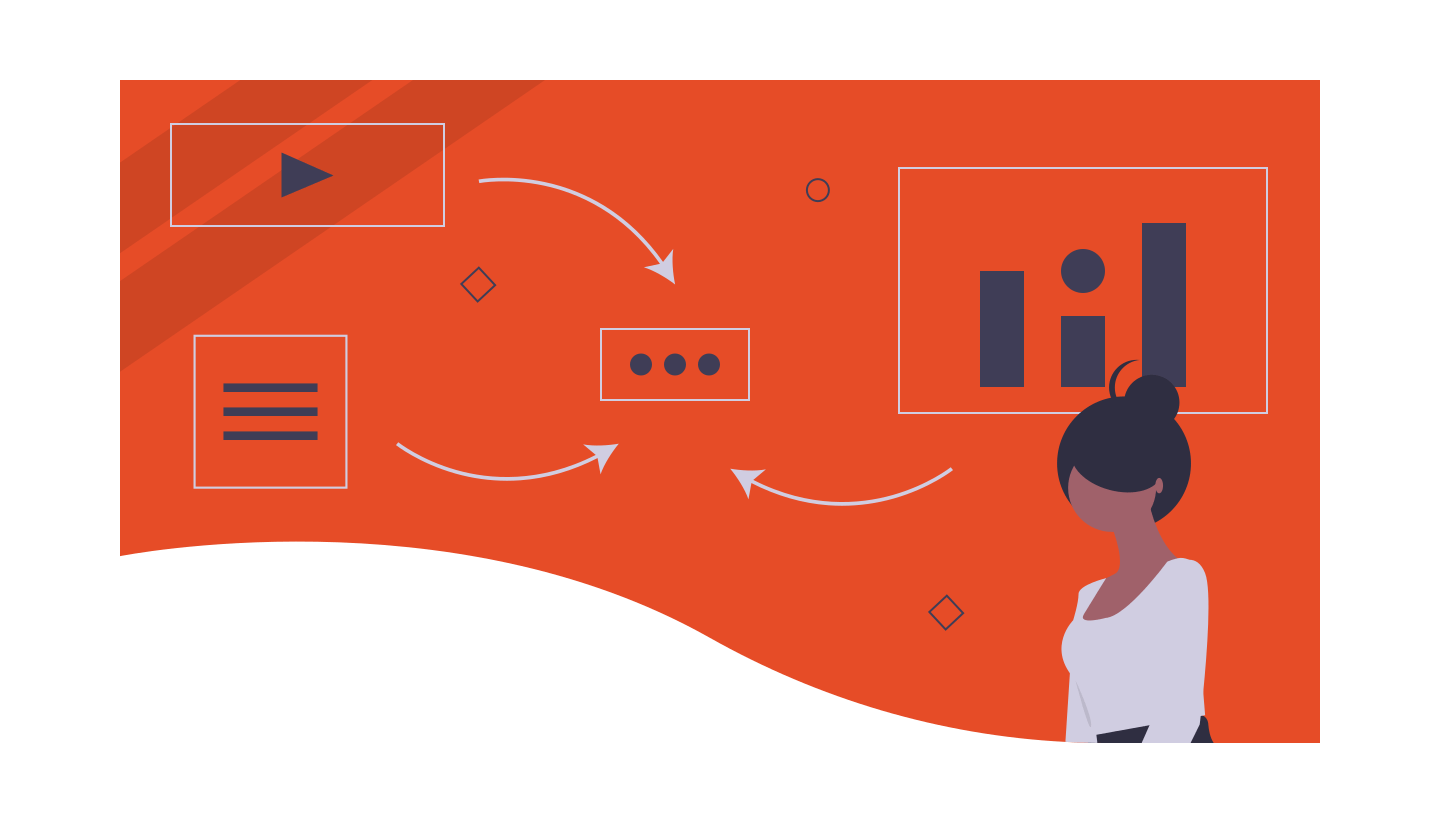One more useful metric is Log-Loss Reduction. This metric is also called reduction in information gain – RIG. It gives a measure of how much a model improves on a model that gives random predictions. Log-loss reduction closer to 1 indicates a better model.

``````var mlContext = new MLContext();

var testSetTransform = trainedModel.Transform(dataSplit.TestSet);

var metrics = mlContext.MulticlassClassification.Evaluate(testSetTransform);

Console.WriteLine(\$"Log Loss: {modelMetrics.LogLoss:#.##}{Environment.NewLine}" +
\$"Log Loss Reduction: {modelMetrics.LogLossReduction:#.##}{Environment.NewLine}");``````
``````Log Loss: .09
Log Loss Reduction: .91``````

## 5. Regression Metrics

Since the goal differs when solving regression problems we need to use different metrics. In general, the output of the regression machine learning model is always continuous and thus metrics need to be aligned for that purpose.

Predictions often deviate from the actual values. Let’s see how we can calculate the quality of these predictions.

### 5.1 Mean Absolute Error – MAE

As the name suggests this metric calculates the average absolute distance (error) between the predicted and target values. It is defined by the formula:Where N represents the number of samples in the dataset, yi is the actual value for the i-th sample, and yi’ is the predicted value for the i-th sample. This metric is robust to outliers, which is really nice. The closer to 0.00, the better quality of predictions. In the code, we use the MeanAbsoluteError of RegressionMetric object:

``````var mlContext = new MLContext();

var testSetTransform = trainedModel.Transform(dataSplit.TestSet);

var metrics = mlContext.MulticlassClassification.Evaluate(testSetTransform);

Console.WriteLine(\$"Mean Absolute Error: {modelMetrics.MeanAbsoluteError:#.##}");``````
``Mean Absolute Error: .65``

### 5.2 Mean Squared Error – MSE

This is probably the most popular metric of all regression metrics. It is quite simple, it finds the average squared distance (error) between the predicted and actual values. The formula that we use to calculate it is:Where N represents the number of samples in the dataset, yi is the actual value for the i-th sample, and yi’ is the predicted value for the ith sample. The result is a non-negative value and the goal is to get this value as close to zero as possible. This function is often used as a loss function of a machine learning model. In the code, we use the MeanSquaredError of a RegressionMetrics object:

``````var mlContext = new MLContext();

var testSetTransform = trainedModel.Transform(dataSplit.TestSet);

var metrics = mlContext.MulticlassClassification.Evaluate(testSetTransform);

Console.WriteLine(\$"Mean Squared Error: {modelMetrics.MeanSquaredError:#.##}");``````
``Mean Squared Error: 1.03``### 5.3 Root Mean Square Error – RMSE

Another very popular metric. It is a variation of the MSE metric. In general, it shows what is the average deviation in predictions from the actual values and it follows an assumption that error is unbiased and follows a normal distribution. We calculate this value by the formula:Just like MSE, RMSE is a non-negative value, and a value 0 is the value we are trying to achieve. A lower RMSE is better than a higher one. In the code, we use RootMeanSquaredError of a RegressionMetrics object.

``````var mlContext = new MLContext();

var testSetTransform = trainedModel.Transform(dataSplit.TestSet);

var metrics = mlContext.MulticlassClassification.Evaluate(testSetTransform);

Console.WriteLine(\$"Root Mean Squared Error: {modelMetrics.RootMeanSquaredError:#.##}");``````
``Root Mean Squared Error: 1.01``

RMSE punishes large errors and is the best metric for large numbers (actual value or prediction). Note that this metric is affected by outliers, so make sure that you remove them from the dataset beforehand.### 5.4 R Squared

The metrics like RMSE and MSE are quite useful, however sometimes not intuitive. What we lack is some sort of benchmark for them. In cases where we need a more intuitive approach, we can use the R-Squared metric. The formula for this metric goes as follows: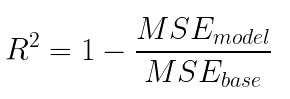Where MSEmodel is the MSE of the predictions against real values, while MSEbase is the MSE of mean prediction against real values. This means that we use the mean of the predictions as a benchmark. Quite elegant, isn’t it? To get this value we use RSquared from RegressionMetrics object:

``````var mlContext = new MLContext();

var testSetTransform = trainedModel.Transform(dataSplit.TestSet);

var metrics = mlContext.MulticlassClassification.Evaluate(testSetTransform);

Console.WriteLine(\$"R Squared: {modelMetrics.RSquared:0.##}");``````
``R Squared: 0.79``

## Conclusion

In this article, we did a very interesting summary of some of the most popular performance metrics that are used in the machine learning world. We separated these metrics into big groups and learned the math behind them and how we can calculate them using ML.NET.

Are you afraid that AI might take your job? Make sure you are the one who is building it.

STAY RELEVANT IN THE RISING AI INDUSTRY! 🖖#### Nikola M. Zivkovic

CAIO at Rubik's Code

Nikola M. Zivkovic a CAIO at Rubik’s Code and the author of book “Deep Learning for Programmers“. He is loves knowledge sharing, and he is experienced speaker. You can find him speaking at meetups, conferences and as a guest lecturer at the University of Novi Sad.

Rubik’s Code is a boutique data science and software service company with more than 10 years of experience in Machine Learning, Artificial Intelligence & Software development. Check out the services we provide.

Ultimate Guide to Machine Learning with Python

Everything from Python basics to the deployment of Machine Learning algorithms to production in one place.

Become a Machine Learning Superhero TODAY!